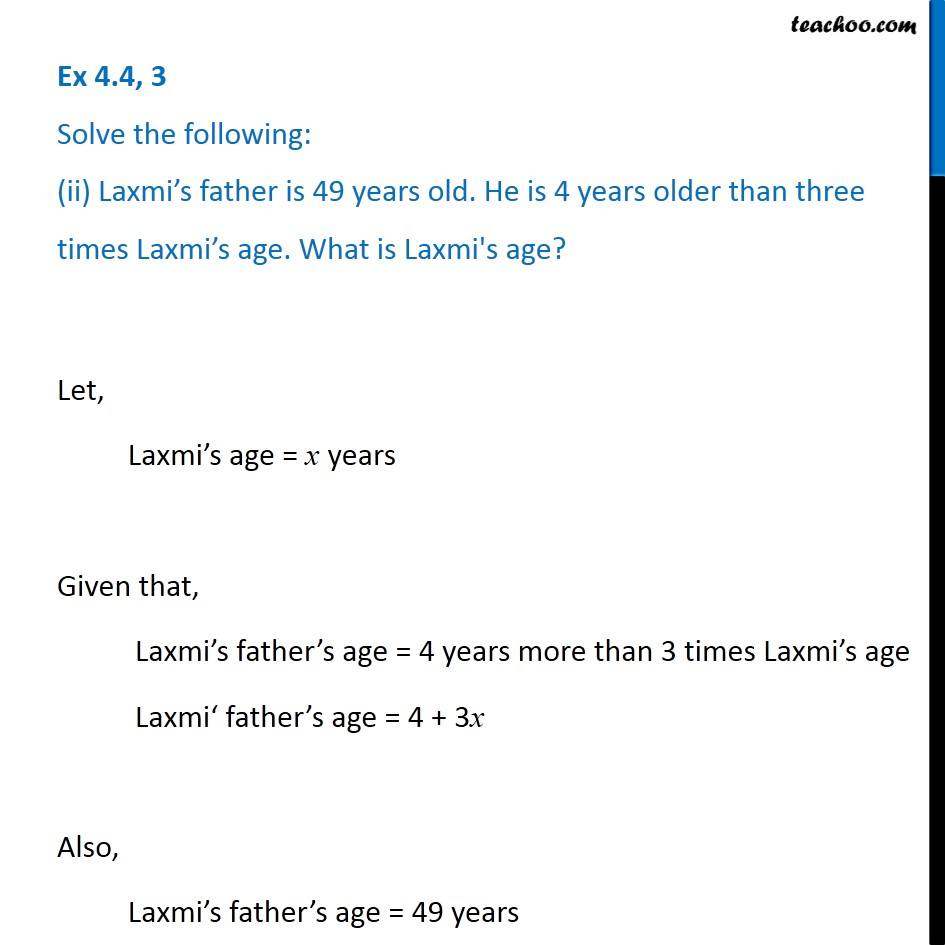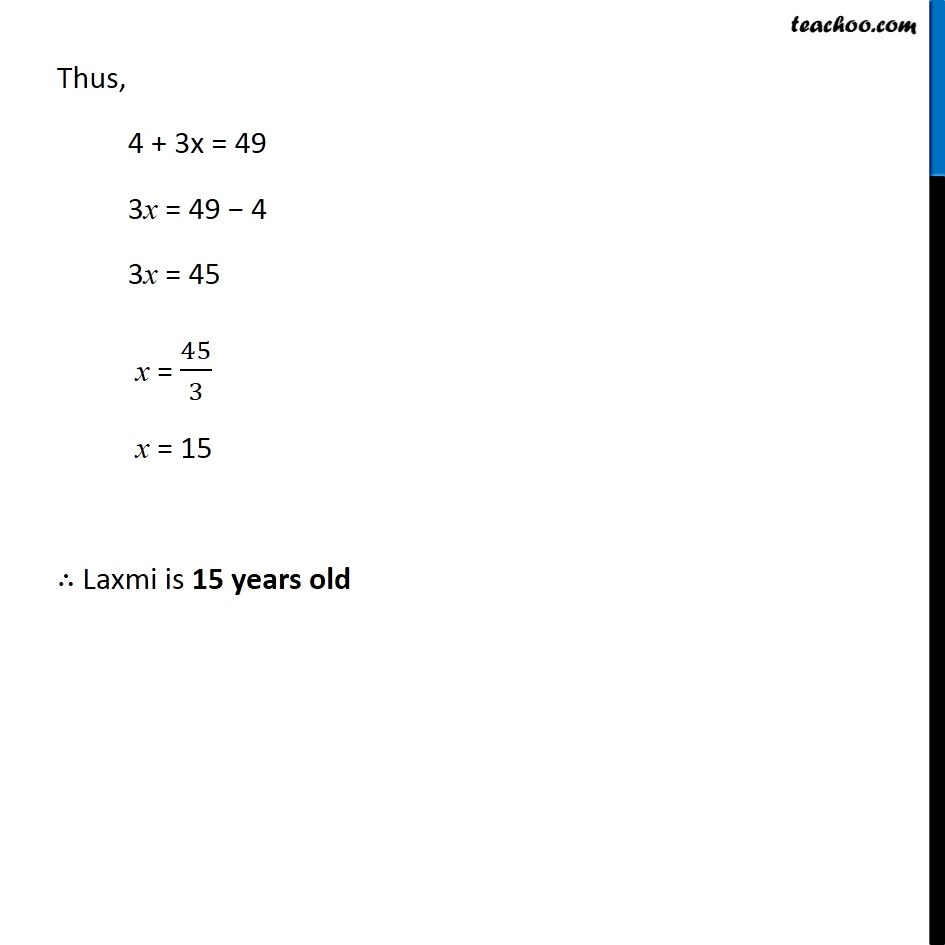Ex 4.4

Chapter 4 Class 7 Simple Equations
Serial order wiseIntroducing your new favourite teacher - Teachoo Black, at only ₹83 per month

### Transcript

Ex 4.4, 3 Solve the following: (ii) Laxmi’s father is 49 years old. He is 4 years older than three times Laxmi’s age. What is Laxmi's age?Let, Laxmi’s age = x years Given that, Laxmi’s father’s age = 4 years more than 3 times Laxmi’s age Laxmi‘ father’s age = 4 + 3x Also, Laxmi’s father’s age = 49 years Thus, 4 + 3x = 49 3x = 49 − 4 3x = 45 x = 45/3 x = 15 ∴ Laxmi is 15 years old